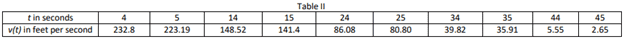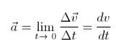# Instantaneous acceleration

• james digel
james digel

## Homework Statement

A. Calculating instantaneous acceleration using formula:

1. According to ‘Table II’ data below:

i. t=5

ii. t=15

iii. t=25

iv. t=35

v. t=45## Homework Equations## The Attempt at a Solution

I wish i understood this topic enough to provide a calculation however I've been spinning my wheels looking at various intro videos and text without being able to apply it successfully to my outcome needed! Thanks all![/B]

#### Attachments

james digel
I've been researching tangent line yet need more guidance about how to properly apply the data set to the equations as I just feel as if I'm missing something crucial I can't place my finger on. Thank you!

Homework Helper
Gold Member
Plot a graph of velocity Vs time. Eg fit a straight line or curve to the data, whichever is appropriate.

Pick a point in time.

Draw a tangent to the curve at that point.

The slope of the tangent is the instantaneous acceleration at that point.

•james digel
Homework Helper
Gold Member
If the fitted line or curve can be described by an equation you can differentiate it to get an equation for the acceleration at all points. Then substitute t with the value at the point of interest.

•james digel
Homework Helper
Gold Member
There are a couple of things to try. If you fit a straight line to your data you get something like ##v(t) = 235.6-5.55t##. This would give you a constant acceleration of -5.55. Alternatively you can estimate the acceleration between any two points by ##\frac{\Delta v}{\Delta t}## and compare results. Taking your first two points you would get ##\frac{223.19-232.8}{5-4}=-9.61##. Try it with other pairs of points and see what you think.

[Edit, added later] Another idea, since the straight line fit isn't too bad, you might just take the straight line through the first and last points and try that. Whatever floats your boat or makes the most sense given the context in which you were given the problem.

Also if you use the ##\frac {\Delta v}{\Delta t}## method you might want to use the alternate point closest to the point where you want the value.

Last edited:
•james digel
Mentor
I've been researching tangent line yet need more guidance about how to properly apply the data set to the equations as I just feel as if I'm missing something crucial I can't place my finger on.
You can't get the tangent line since you are not given the formula for the function that generates the (t, v(t)) pairs of data. What the problem is asking you to do is to approximate the slope of the tangent line by a secant line. A secant line is the segment between two points on a curve; i.e., between ##(t_i, v(t_i))## and ##(t_j, v(t_j))##. To approximate acceleration at t = 5, the best results will most likely come from using the data on either side. IOW, the data for t = 4 and t = 14.

And so on, for the other times in the problem.

The crucial pieces you're missing are the concepts of tangent line and secant line. Your textbook must have a discussion of these concepts, so read up on them.

•james digel
james digel
Thank you, Mark44, LCKurtz, and Cwatters! I appreciate all the help I got a good 12 hours of sleep now back at it!